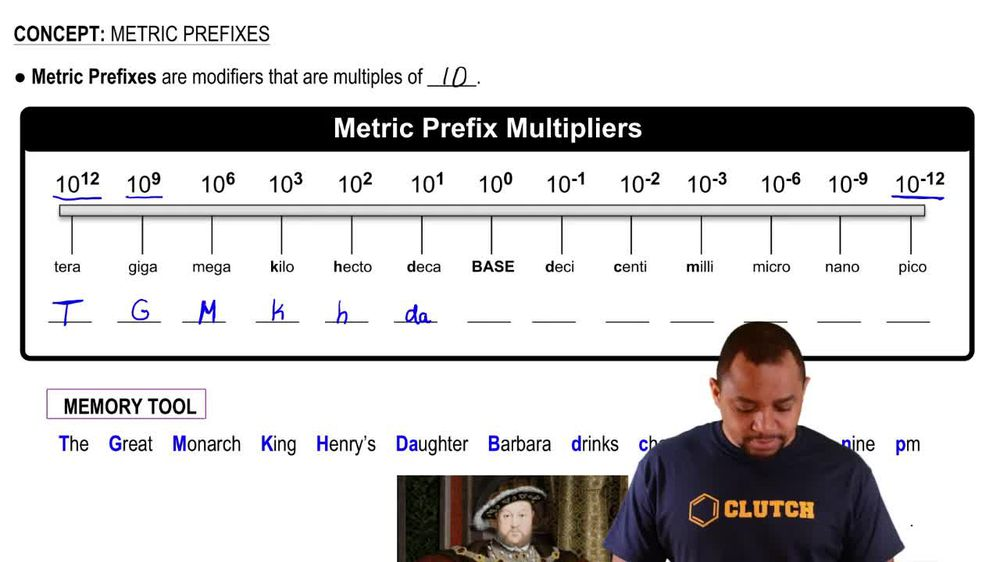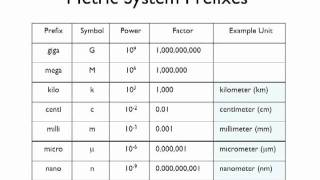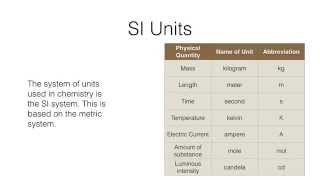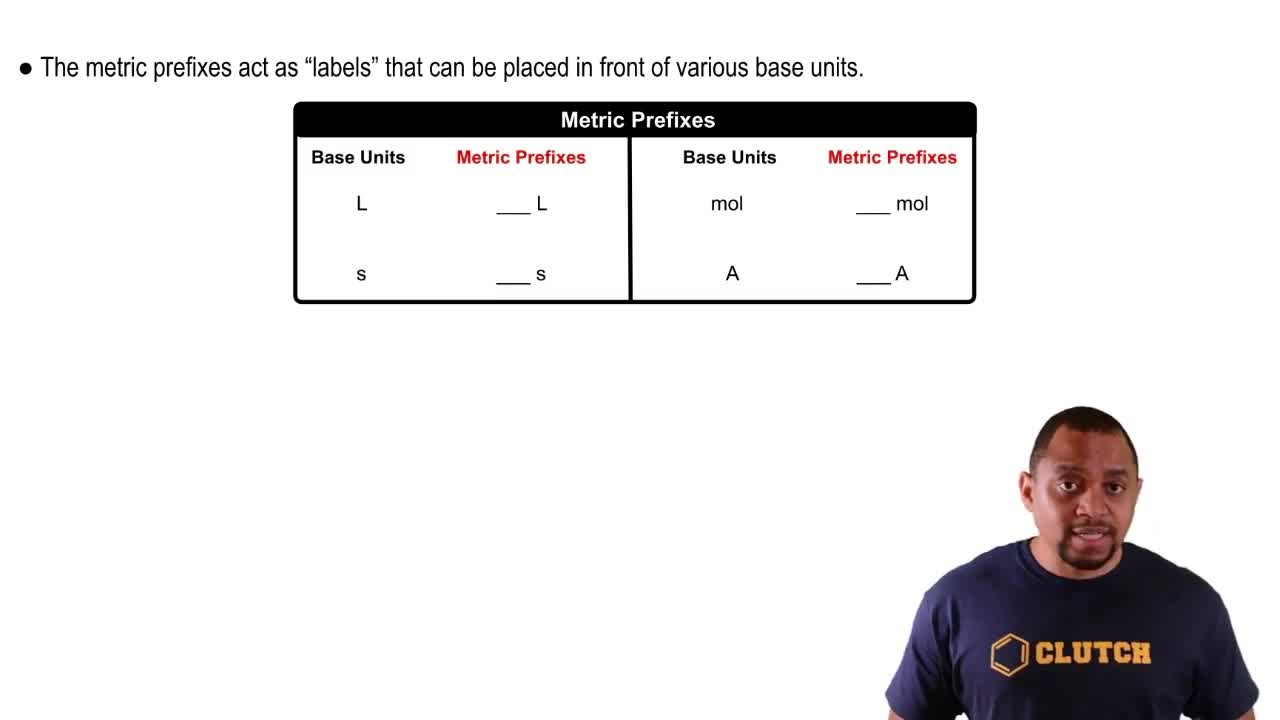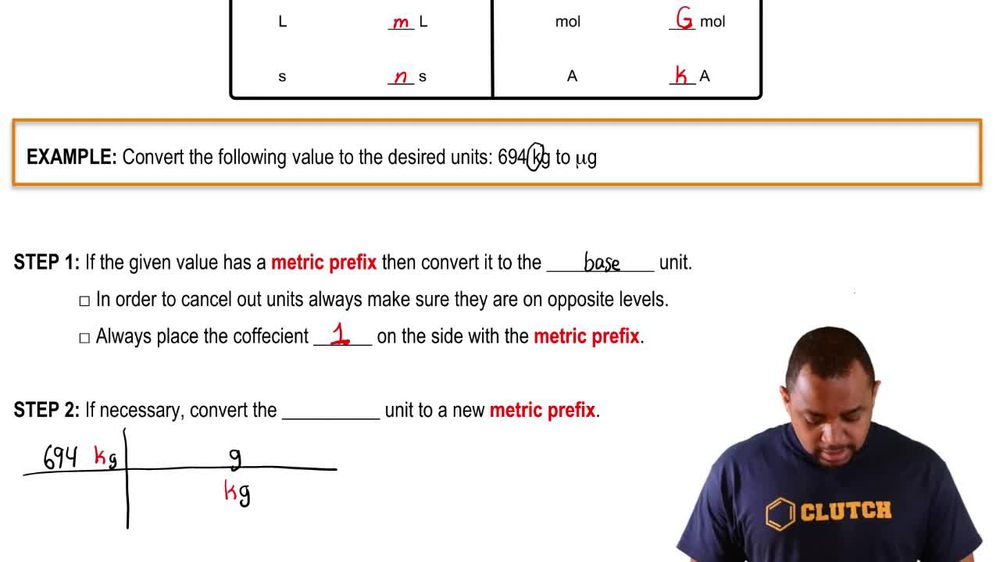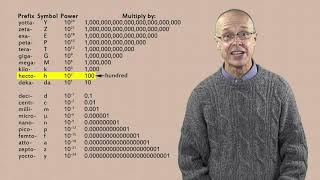Start typing, then use the up and down arrows to select an option from the list.
1. 1. Intro to General Chemistry2. Metric Prefixes
Problem

# Complete the table. a. 1245 kg 1.245 * 106 g 1.245 * 109 mg b. 515 km _____dm _____cm c. 122.355 s _____ms _____ks d. 3.345 kJ _____J _____mJ

Relevant Solution1m
Play a video:
Hi everyone today, we have a question asking us to use scientific notation and write the following measurements without prefix multipliers. 2.5 kg. So we have to remember that one kg Equals 10 to the 3rd g. And now we can put this in an equation. So we have 2.5 kg. We're going to multiply that by 10 to 3rd g over one kg. And our kg will cancel out here, leaving us with g and that's going to equal 2500. Now we want to put it into scientific notation, so we're going to take our decimal .1 2 3 places to the left, Which makes it 2.5 times cubed. And that is our answer. Thank you for watching. Bye.